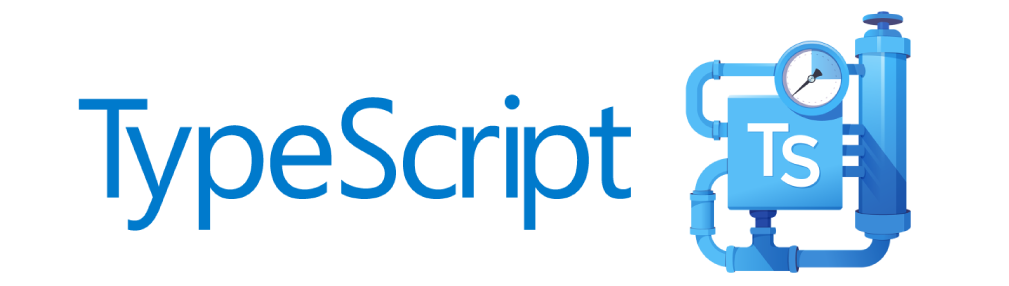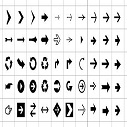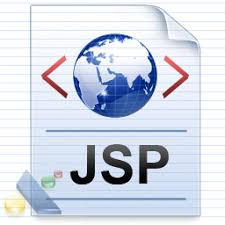# Oracle ANYDATA Type Usage in SQL,PL/SQL and Complex Types

The ANYDATA type was first introduced in Oracle 9i. It is a self describing type, containing an instance of a given type along with a description of the type it contains. With the exception of BLOB and CLOB types, ANYDATA types can be persisted in database tables.

## SQL

The ANYDATA type includes a number of member functions to manipulate the contents from SQL and PL/SQL. Create the following table to run the examples below.

```DROP TABLE anydata_test;

CREATE TABLE anydata_test (
id      NUMBER,
content SYS.ANYDATA
);
```

The ANYDATA type includes CONVERT* constructor functions for the majority of Oracle data types that can be accessed from SQL. The code below uses some of them, but a full list can be found here.

```INSERT INTO anydata_test (id, content) VALUES (1, SYS.ANYDATA.convertVarchar2('This is some data'));
INSERT INTO anydata_test (id, content) VALUES (2, SYS.ANYDATA.convertNumber(1234567890));
INSERT INTO anydata_test (id, content) VALUES (3, SYS.ANYDATA.convertDate(TO_DATE('01-JAN-2012','DD-MON-YYYY')));
COMMIT;
```

The GETTYPENAME function displays the type of data contained in a specific ANYDATA type.

```COLUMN type_name FORMAT A20

SELECT id,
SYS.ANYDATA.getTypeName(content) AS type_name
FROM   anydata_test
ORDER BY id;

ID TYPE_NAME
---------- --------------------
1 SYS.VARCHAR2
2 SYS.NUMBER
3 SYS.DATE

3 rows selected.

SQL>
```

This can be used to determine how to process the data. The following example uses a combination of the GETTYPENAME and ACCESS* functions to process and output the data stored in the table. A full list of supported ACCESS* functions can be found here.

```COLUMN content FORMAT A20

SELECT id,
(CASE SYS.ANYDATA.getTypeName(content)
WHEN 'SYS.VARCHAR2' THEN SYS.ANYDATA.accessVarchar2(content)
WHEN 'SYS.NUMBER'   THEN TO_CHAR(SYS.ANYDATA.accessNumber(content))
WHEN 'SYS.DATE'     THEN TO_CHAR(SYS.ANYDATA.accessDate(content), 'DD-MON-YYYY')
END) AS content
FROM   anydata_test;

ID CONTENT
---------- --------------------
1 This is some data
2 1234567890
3 01-JAN-2012

3 rows selected.

SQL>
```

## PL/SQL

The CONVERT*, ACCESS* and GETTYPENAME functions are also available from PL/SQL. Since the following example doesn't persist anything to the database, it also includes BLOB and CLOB conversions.

```SET SERVEROUTPUT ON

DECLARE
l_varchar2 VARCHAR2(50) := 'This is some data';
l_number   NUMBER       := 1234567890;
l_date     DATE         := TO_DATE('01-JAN-2012','DD-MON-YYYY');
l_clob     CLOB         := 'This is some CLOB data';
l_blob     BLOB         := UTL_RAW.cast_to_raw('This is some BLOB data');
l_anydata  SYS.ANYDATA;
BEGIN
-- Convert VARCHAR2 to ANYDATA and back.
l_anydata  := SYS.ANYDATA.convertVarchar2(l_varchar2);
l_varchar2 := SYS.ANYDATA.accessVarchar2(l_anydata);
DBMS_OUTPUT.put_line('VARCHAR2: ' || l_varchar2);

-- Convert NUMBER to ANYDATA and back.
l_anydata  := SYS.ANYDATA.convertNumber(l_number);
l_number   := SYS.ANYDATA.accessNumber(l_anydata);
DBMS_OUTPUT.put_line('NUMBER  : ' || TO_CHAR(l_number));

-- Convert DATE to ANYDATA and back.
l_anydata  := SYS.ANYDATA.convertDate(l_date);
l_date     := SYS.ANYDATA.accessDate(l_anydata);
DBMS_OUTPUT.put_line('DATE    : ' || TO_CHAR(l_date, 'DD-MON-YYYY'));

-- Convert ANYDATA to CLOB
l_anydata  := SYS.ANYDATA.convertClob(l_clob);
l_clob     := SYS.ANYDATA.accessClob(l_anydata);
DBMS_OUTPUT.put_line('CLOB    : ' || l_clob);

-- Convert ANYDATA to BLOB
l_anydata  := SYS.ANYDATA.convertBlob(l_blob);
l_blob     := SYS.ANYDATA.accessBlob(l_anydata);
DBMS_OUTPUT.put_line('BLOB    : ' || UTL_RAW.cast_to_varchar2(l_blob));
END;
/
VARCHAR2: This is some data
NUMBER  : 1234567890
DATE    : 01-JAN-2012
CLOB    : This is some CLOB data
BLOB    : This is some BLOB data

PL/SQL procedure successfully completed.

SQL>
```

From PL/SQL we can also use the GET* functions to pull the data out of an ANYDATA type into a variable.

```SET SERVEROUTPUT ON

DECLARE
l_varchar2 VARCHAR2(50) := 'This is some data';
l_anydata  SYS.ANYDATA;
BEGIN
-- Convert VARCHAR2 to ANYDATA and back.
l_anydata  := SYS.ANYDATA.convertVarchar2(l_varchar2);

IF l_anydata.getVarchar2(l_varchar2) = DBMS_TYPES.SUCCESS THEN
DBMS_OUTPUT.put_line('VARCHAR2: ' || l_varchar2);
END IF;
END;
/
VARCHAR2: This is some data

PL/SQL procedure successfully completed.

SQL>
```

## Complex Types

Complex object types and user-defined objects types can also be serialized as ANYDATA types. This is done using the CONVERTOBJECT and GETOBJECT functions.

```CREATE OR REPLACE TYPE t_my_type AS OBJECT (
g_val1 VARCHAR2(10),
g_val2 VARCHAR2(10)
);
/
l_obj     t_my_type := t_my_type('1111111111', '2222222222');
l_anydata SYS.ANYDATA;
BEGIN
-- Convert Object to ANYDATA and back.
l_anydata := SYS.ANYDATA.convertObject(l_obj);

IF l_anydata.getObject(l_obj) = DBMS_TYPES.SUCCESS THEN
DBMS_OUTPUT.put_line('T_MY_TYPE : ' || l_obj.g_val1 || ' : ' || l_obj.g_val2);
END IF;
END;
/
T_MY_TYPE : 1111111111 : 2222222222

PL/SQL procedure successfully completed.

SQL>
```Admin on Apr 20, 2019 at 12:01 am

If you like dEexams.com and would like to contribute, you can write your article here or mail your article to admin@deexams.com . See your article appearing on the dEexams.com main page and help others to learn.

#### Post Comment

##### WEB TECHNOLOGY##### TypeScript Programmning Language##### New HTML5 APIs##### HTML Arrow -> Range: Decimal 8592-8703. Hex 2190-21FF.##### How To Override One CSS Class With Another×